# Celebration of 60 years old birthdays of Prof

• Slides: 29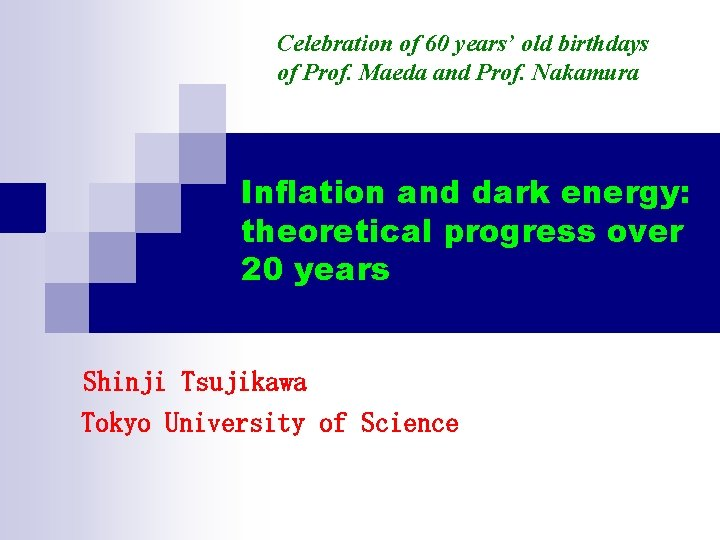Celebration of 60 years’ old birthdays of Prof. Maeda and Prof. Nakamura Inflation and dark energy: theoretical progress over 20 years Shinji Tsujikawa 　 Tokyo University of Science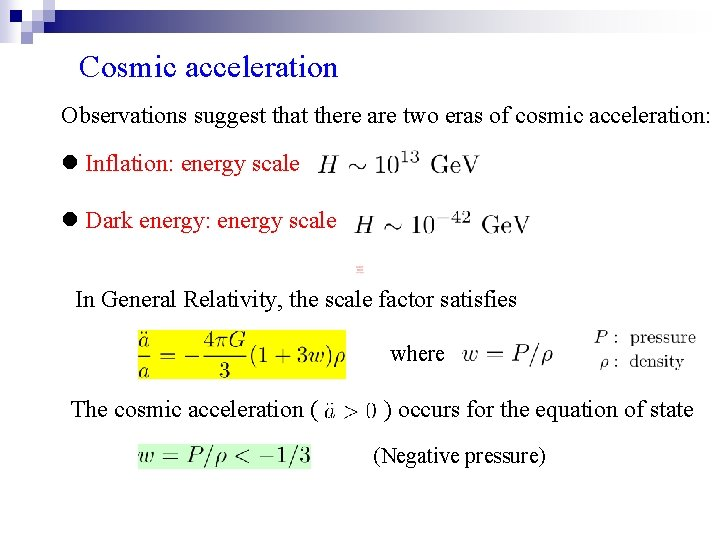Cosmic acceleration Observations suggest that there are two eras of cosmic acceleration: l Inflation: energy scale l Dark energy: energy scale In General Relativity, the scale factor satisfies where The cosmic acceleration ( ) occurs for the equation of state (Negative pressure)Original papers of inflation The idea of inflation was proposed by several people independently. l Curvature inflation l. Starobinsky, Phys. Lett. B 91, 99 (1980) The higher-order curvature term leads to inflation. l “Old’’ inflation Sato, Mon. Not. R. Astron. Soc. 195, 467 (1981) Kazanas, Astrophys. J. Lett. 241, L 59 (1980) Guth, Phys. Rev. D 23, 347 (1981) Inflation occurs due to the first-order phase transition of a vacuum.Inflation based on Grand unified theories Inflationary universe: A possible solution to the horizon and flatness problems Alan H. Guth* Stanford Linear Accelerator Center, Stanford University, Stanford, California 94305 ______ 472 citations _______ Received 11 August 1980 3641 citations 135 citations ________________________So far many inflation models have been proposed. Most of them are based on a scalar field with a potential. f(R), old, new, chaotic, extended, power-law, hybrid, natural, supernatural, extra-natural, eternal, D-term, F-term, brane, oscillating, tachyon, dilaton, Quintessenatial, K-inflation, ghost condensate, KKLMMT, DBI, hill-top, Higgs, Galileon, …. Over 3300 papers in which the titles include the word “inflation’’.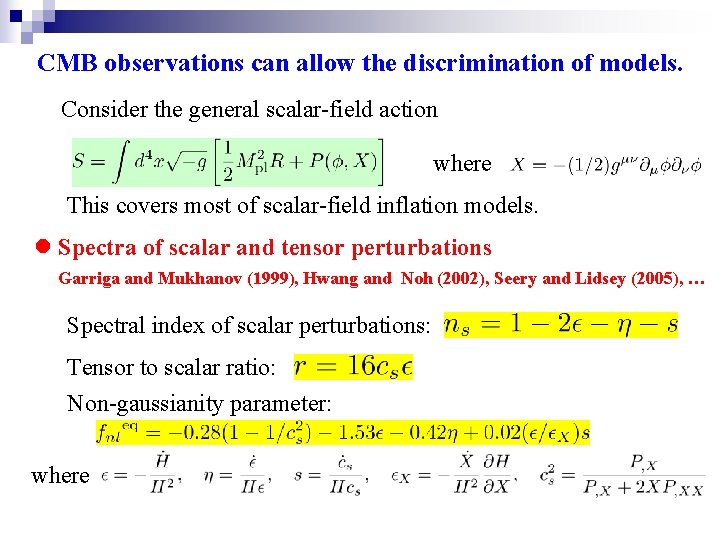CMB observations can allow the discrimination of models. Consider the general scalar-field action where This covers most of scalar-field inflation models. l Spectra of scalar and tensor perturbations Garriga and Mukhanov (1999), Hwang and Noh (2002), Seery and Lidsey (2005), … Spectral index of scalar perturbations: Tensor to scalar ratio: Non-gaussianity parameter: where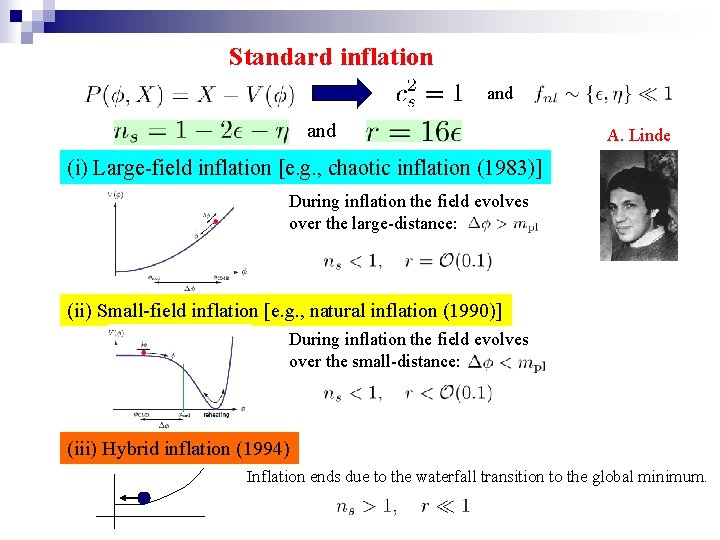Standard inflation and A. Linde (i) Large-field inflation [e. g. , chaotic inflation (1983)] During inflation the field evolves over the large-distance: (ii) Small-field inflation [e. g. , natural inflation (1990)] During inflation the field evolves over the small-distance: (iii) Hybrid inflation (1994) Inflation ends due to the waterfall transition to the global minimum.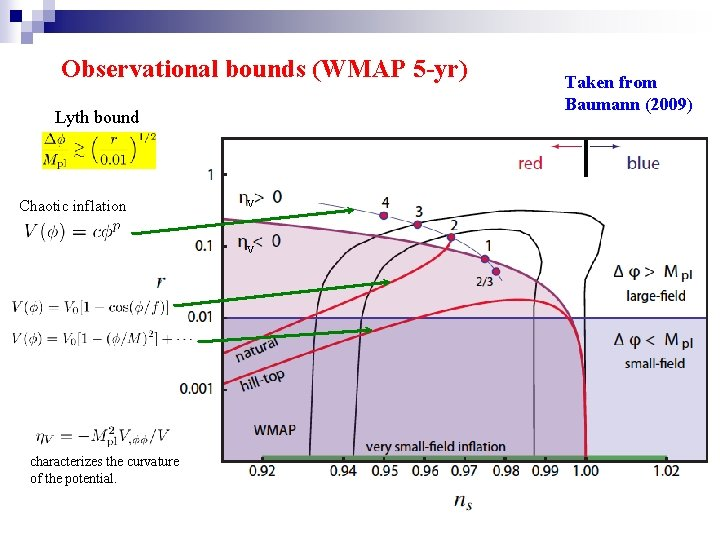Observational bounds (WMAP 5 -yr) Lyth bound Chaotic inflation V V characterizes the curvature of the potential. Taken from Baumann (2009)Kinetic inflation Kinetic-type inflation appears in many contexts: (i) Low energy effective string theory (Armendariz-Picon, Damour, Mukhanov, 1999) (ii) DBI theories (Silverstein and Tong, 2004) (iii) Ghost condensate (Arkani-Hamed et al, 2004) (iv) Galileon gravity: (Nicolis et al, 2008) The Lagrangian is constructed to satisfy the Galilean symmetry in flat space-time, e. g. , Kobayashi, Yamaguchi, Yokoyama (2010), Or its generalization: Mizuno and Koyama (2010) Testable in Planck satteliteStarobinsky’s inflation model (modified gravity model) See K. Maeda, PRD 37: 858 (1988) for the analysis in Bianchi specetime. The slow-roll parameter is (quasi-exponential expansion) Inflation ends around For this model the scalar spectral index and the tensor-to-scalar ratio are The number of e-foldings from the end of inflation These values satisfy the WMAP bounds: Starobinksy (1983) Kofman et al (1987) Hwang and Noh (2001), …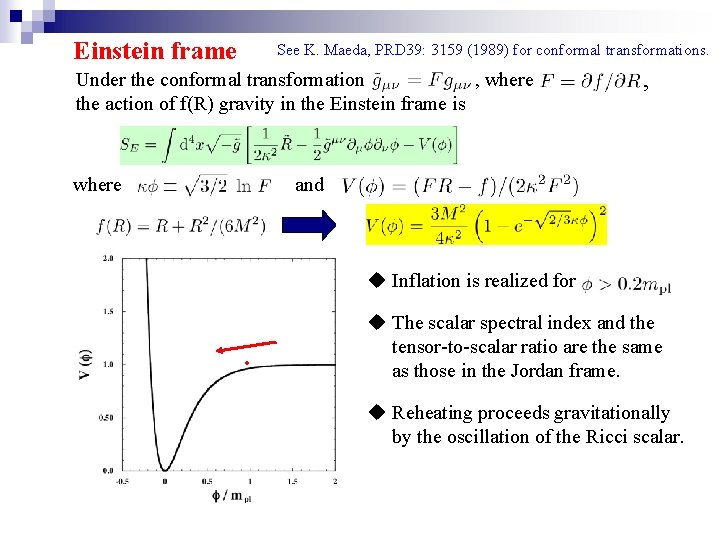Einstein frame See K. Maeda, PRD 39: 3159 (1989) for conformal transformations. Under the conformal transformation , where , the action of f(R) gravity in the Einstein frame is where and u Inflation is realized for . u The scalar spectral index and the tensor-to-scalar ratio are the same as those in the Jordan frame. u Reheating proceeds gravitationally by the oscillation of the Ricci scalar.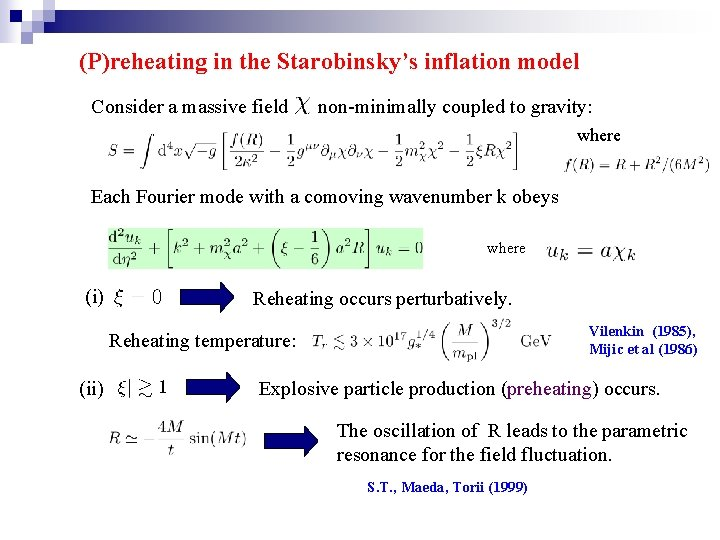(P)reheating in the Starobinsky’s inflation model Consider a massive field non-minimally coupled to gravity: where Each Fourier mode with a comoving wavenumber k obeys where (i) Reheating occurs perturbatively. Vilenkin (1985), Mijic et al (1986) Reheating temperature: (ii) Explosive particle production (preheating) occurs. The oscillation of R leads to the parametric resonance for the field fluctuation. S. T. , Maeda, Torii (1999)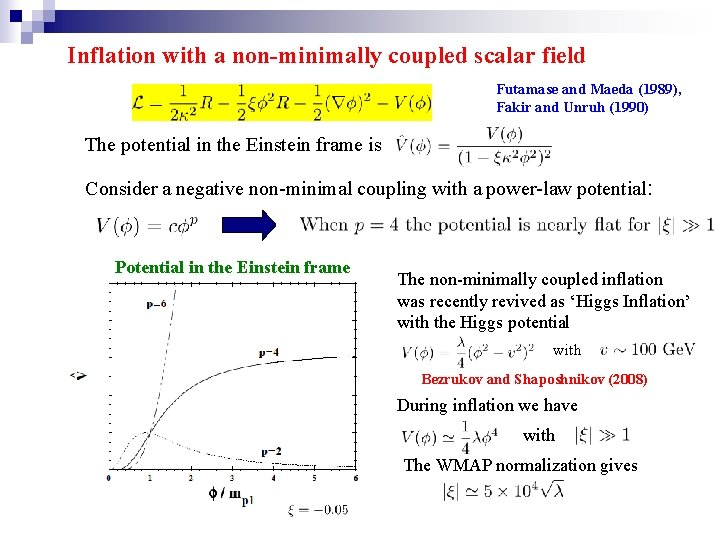Inflation with a non-minimally coupled scalar field Futamase and Maeda (1989), Fakir and Unruh (1990) The potential in the Einstein frame is Consider a negative non-minimal coupling with a power-law potential: Potential in the Einstein frame The non-minimally coupled inflation was recently revived as ‘Higgs Inflation’ with the Higgs potential with Bezrukov and Shaposhnikov (2008) During inflation we have with The WMAP normalization gives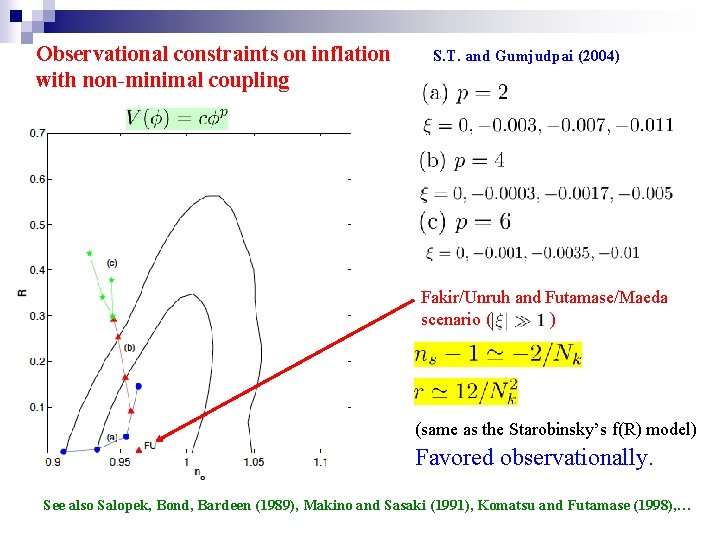Observational constraints on inflation with non-minimal coupling S. T. and Gumjudpai (2004) Fakir/Unruh and Futamase/Maeda scenario ( ) (same as the Starobinsky’s f(R) model) Favored observationally. See also Salopek, Bond, Bardeen (1989), Makino and Sasaki (1991), Komatsu and Futamase (1998), …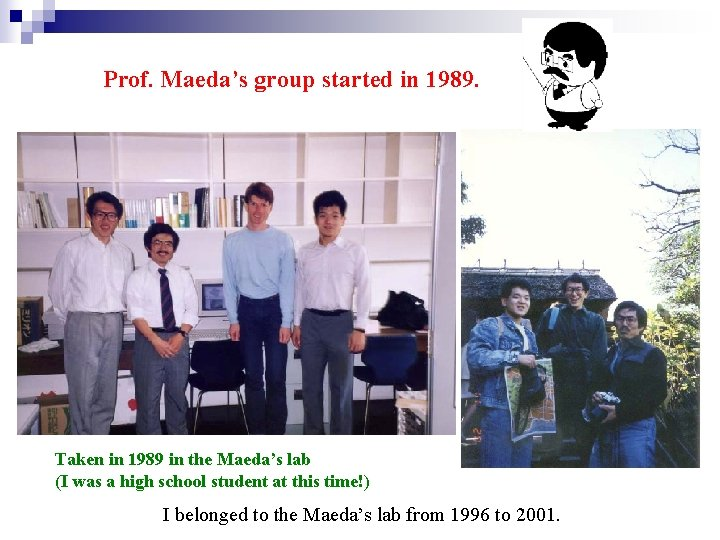Prof. Maeda’s group started in 1989. Taken in 1989 in the Maeda’s lab (I was a high school student at this time!) I belonged to the Maeda’s lab from 1996 to 2001.Dark Energy (1998~) Perlmutter et al, Riess et al (1998) From Supernovae observations they showed that the cosmological constant ( ) exists at the 99 % confidence level. Matter ratio today： (Perlmutter et al, 1998) About 70 % is dark energy responsible for the cosmic acceleration today. High-z data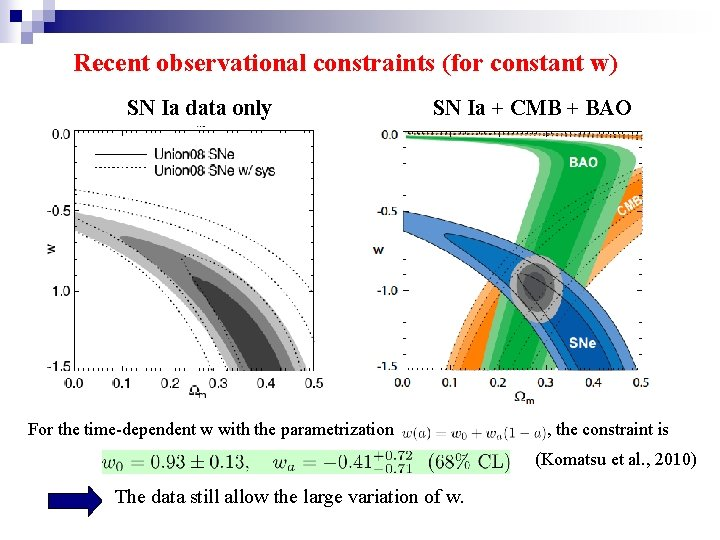Recent observational constraints (for constant w) SN Ia data only SN Ia + CMB + BAO For the time-dependent w with the parametrization , the constraint is (Komatsu et al. , 2010) The data still allow the large variation of w.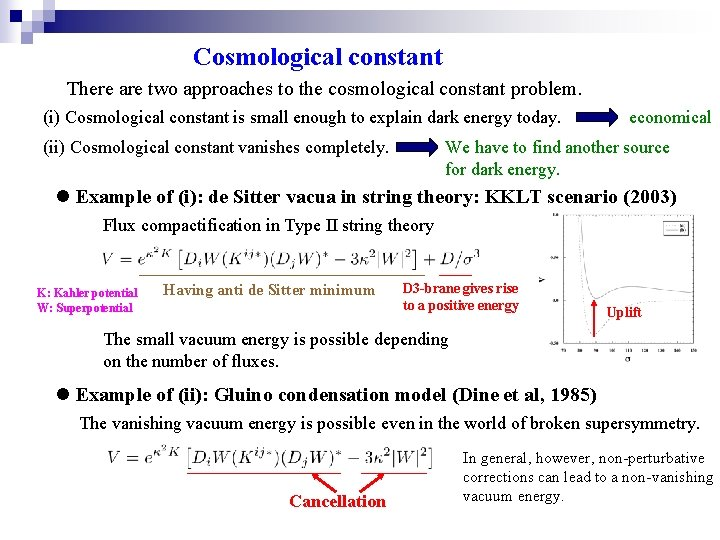Cosmological constant There are two approaches to the cosmological constant problem. (i) Cosmological constant is small enough to explain dark energy today. (ii) Cosmological constant vanishes completely. economical We have to find another source for dark energy. l Example of (i): de Sitter vacua in string theory: KKLT scenario (2003) Flux compactification in Type II string theory _____________ ___ K: Kahler potential W: Superpotential Having anti de Sitter minimum D 3 -brane gives rise to a positive energy Uplift The small vacuum energy is possible depending on the number of fluxes. l Example of (ii): Gluino condensation model (Dine et al, 1985) The vanishing vacuum energy is possible even in the world of broken supersymmetry. _______ Cancellation In general, however, non-perturbative corrections can lead to a non-vanishing vacuum energy.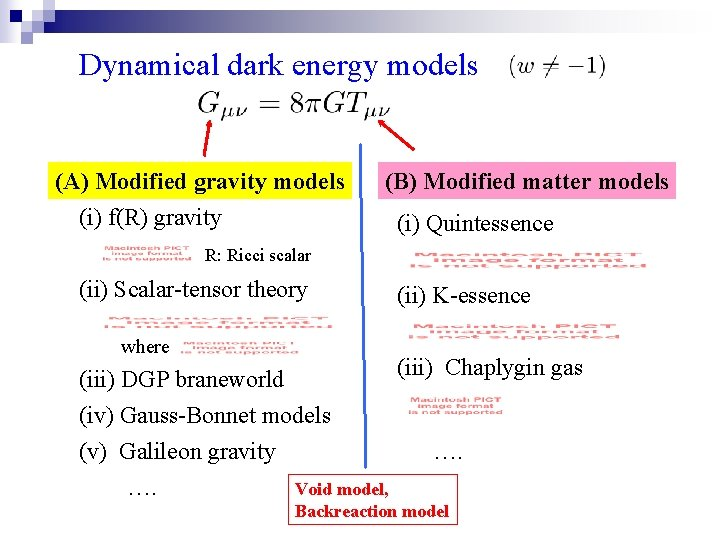Dynamical dark energy models (A) Modified gravity models (i) f(R) gravity (B) Modified matter models (i) Quintessence R: Ricci scalar (ii) Scalar-tensor theory (ii) K-essence where (iii) Chaplygin gas (iii) DGP braneworld (iv) Gauss-Bonnet models (v) Galileon gravity …. …. Void model, Backreaction model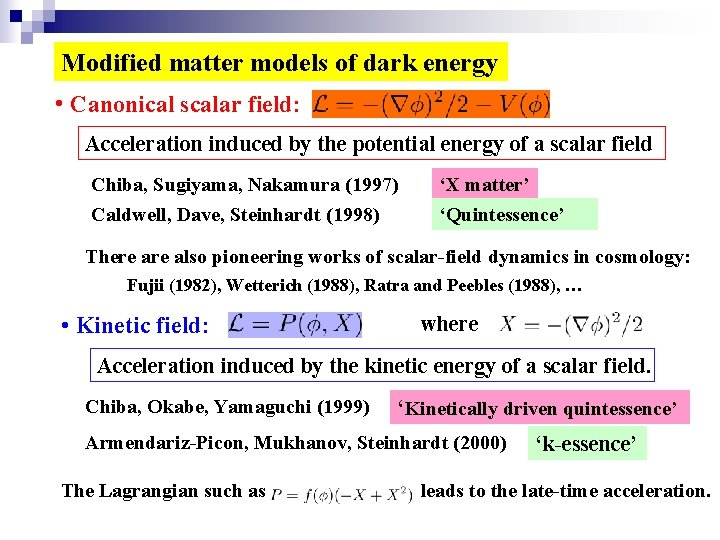Modified matter models of dark energy • Canonical scalar field: Acceleration induced by the potential energy of a scalar field Chiba, Sugiyama, Nakamura (1997) Caldwell, Dave, Steinhardt (1998) ‘X matter’ ‘Quintessence’ There also pioneering works of scalar-field dynamics in cosmology: Fujii (1982), Wetterich (1988), Ratra and Peebles (1988), … • Kinetic field: where Acceleration induced by the kinetic energy of a scalar field. Chiba, Okabe, Yamaguchi (1999) ‘Kinetically driven quintessence’ Armendariz-Picon, Mukhanov, Steinhardt (2000) The Lagrangian such as ‘k-essence’ leads to the late-time acceleration.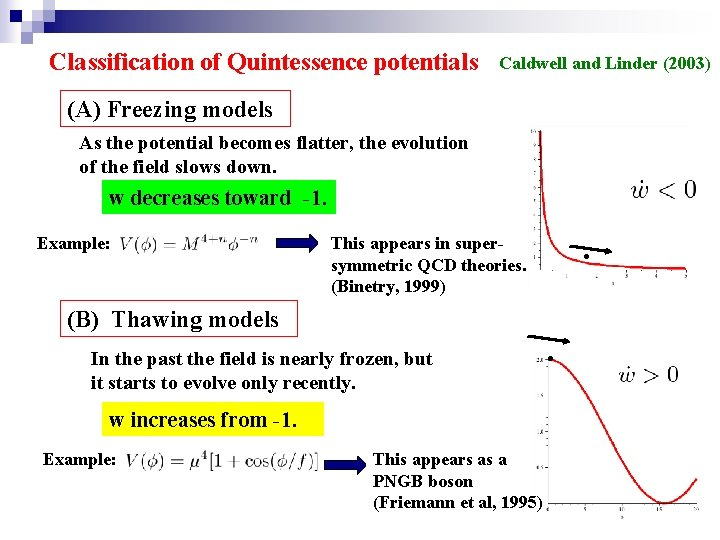Classification of Quintessence potentials Caldwell and Linder (2003) (A) Freezing models As the potential becomes flatter, the evolution of the field slows down. w decreases toward -1. Example: (B) Thawing models In the past the field is nearly frozen, but it starts to evolve only recently. w increases from -1. Example: . This appears in supersymmetric QCD theories. (Binetry, 1999) This appears as a PNGB boson (Friemann et al, 1995) .Particle physics models of quintessence To explain the cosmic acceleration today, the mass of the field is required to be very light. l In particle physics models, the flatness of the potential may be spoiled by radiative corrections to the potential (Kolda and Lyth, 1999). l Such a light scalar field may interact with standard model particles (Carroll, 1999). Good model based on particle physics Pseudo-Nambu-Goldston model (axion field) See e. g. , Kim ane Nilles (2003) Hall, Nomura, Oliver (2005) The small mass can be protected against radiative corrections.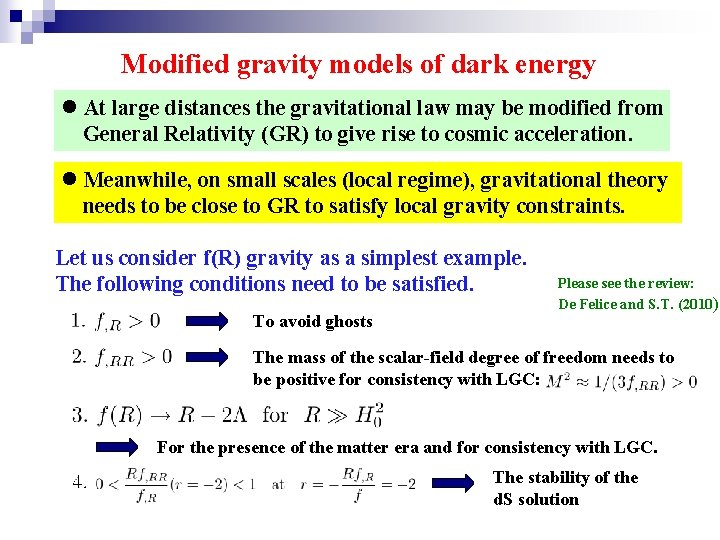Modified gravity models of dark energy l At large distances the gravitational law may be modified from General Relativity (GR) to give rise to cosmic acceleration. l Meanwhile, on small scales (local regime), gravitational theory needs to be close to GR to satisfy local gravity constraints. Let us consider f(R) gravity as a simplest example. The following conditions need to be satisfied. To avoid ghosts Please see the review: De Felice and S. T. (2010) The mass of the scalar-field degree of freedom needs to be positive for consistency with LGC: For the presence of the matter era and for consistency with LGC. The stability of the d. S solutionViable f(R) dark energy models (n>0) Hu and Sawicki, 2007 Starobinsky, 2007 S. T. , 2007 These satisfy f(R=0)=0. Cosmological constant disappears in flat space-time. R is roughly of the order of the present cosmological Ricci scalar. c The models approach the LCDM for . (for the models 1 and 2) The local gravity constraints can be satisfied for (Capozziello and S. T. , 2008)Chameleon mechanism in f(R) dark energy models Viable f(R) models have been constructed to satisfy local gravity constraints in the regions of high density. Potential in Einstein frame for the Starobinsky’s model l Frolov (2008), Kobayashi and Maeda (2008) Massive (The field does not propagate freely) Massless Relativistic stars may be difficult to exist because of the singularity. l S. T. , Tamaki, Tavakol (2009) Upadhye and Hu (2009) Babichev and Langlois (2009) Relativistic stars exist, while the boundary conditions need to be chosen carefully. The effective potential is _______ Matter coupling l For general varying density stars De Felice and Maeda, in preparation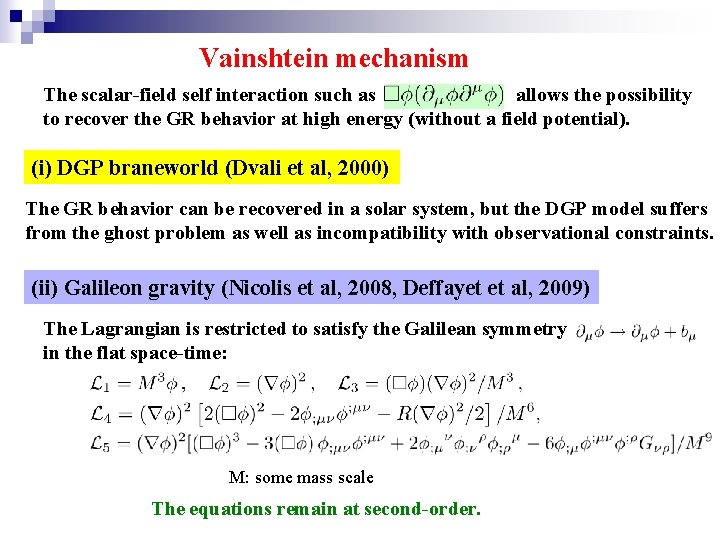Vainshtein mechanism The scalar-field self interaction such as allows the possibility to recover the GR behavior at high energy (without a field potential). (i) DGP braneworld (Dvali et al, 2000) The GR behavior can be recovered in a solar system, but the DGP model suffers from the ghost problem as well as incompatibility with observational constraints. (ii) Galileon gravity (Nicolis et al, 2008, Deffayet et al, 2009) The Lagrangian is restricted to satisfy the Galilean symmetry in the flat space-time: M: some mass scale The equations remain at second-order.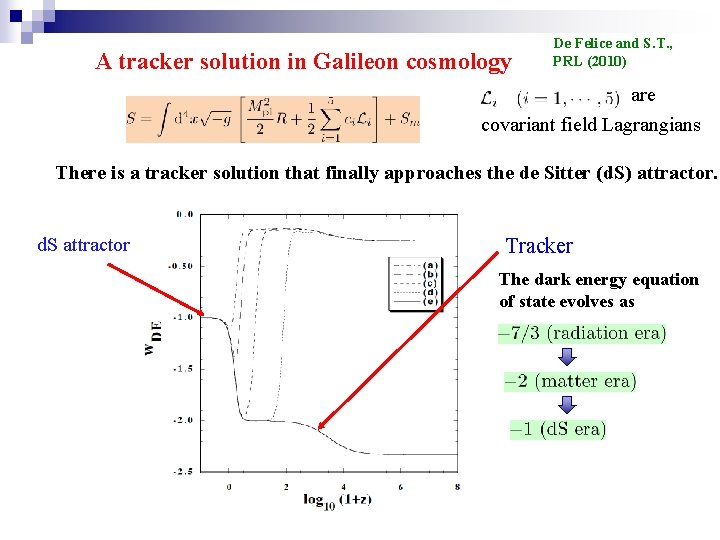A tracker solution in Galileon cosmology De Felice and S. T. , PRL (2010) are covariant field Lagrangians There is a tracker solution that finally approaches the de Sitter (d. S) attractor. d. S attractor Tracker The dark energy equation of state evolves asSummary of dark energy models (1) Cosmological constant (w= -1) Observationally, good. Theoretically, further progress is required. (2) Modified matter models Quintessence, k-essence: So far these are not distinguished from LCDM observationally. Chaplygin gas: × Ruled out from observations of large-scale structure. (3) Modified gravity models f(R) gravity, scalar-tensor theory: Possible to design viable models. DGP braneworld： × Gauss-Bonnet gravity: ( and Ruled out from observations and ghost problem. × Ruled out from the instability of perturbations. ) Galieon gravity: The analysis of density perturbations is required to test this model.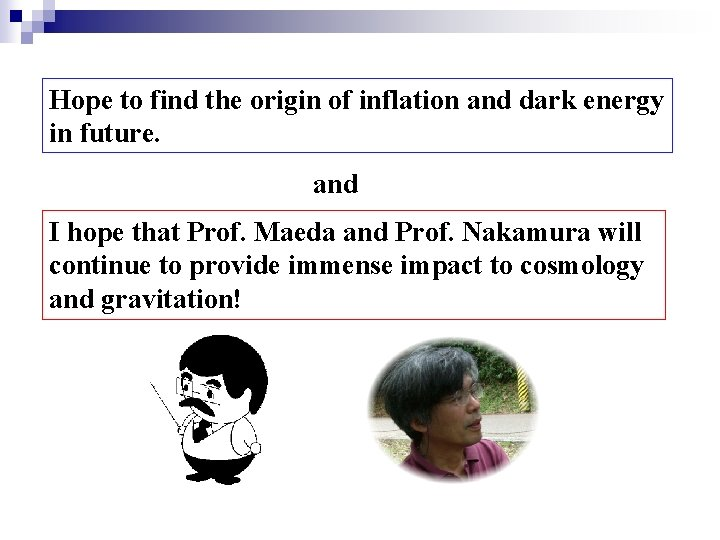Hope to find the origin of inflation and dark energy in future. and I hope that Prof. Maeda and Prof. Nakamura will continue to provide immense impact to cosmology and gravitation!